Intro to Perimeter Math Videos for Kids - 3rd, 4th, & 5th Grades
1%
It was processed successfully!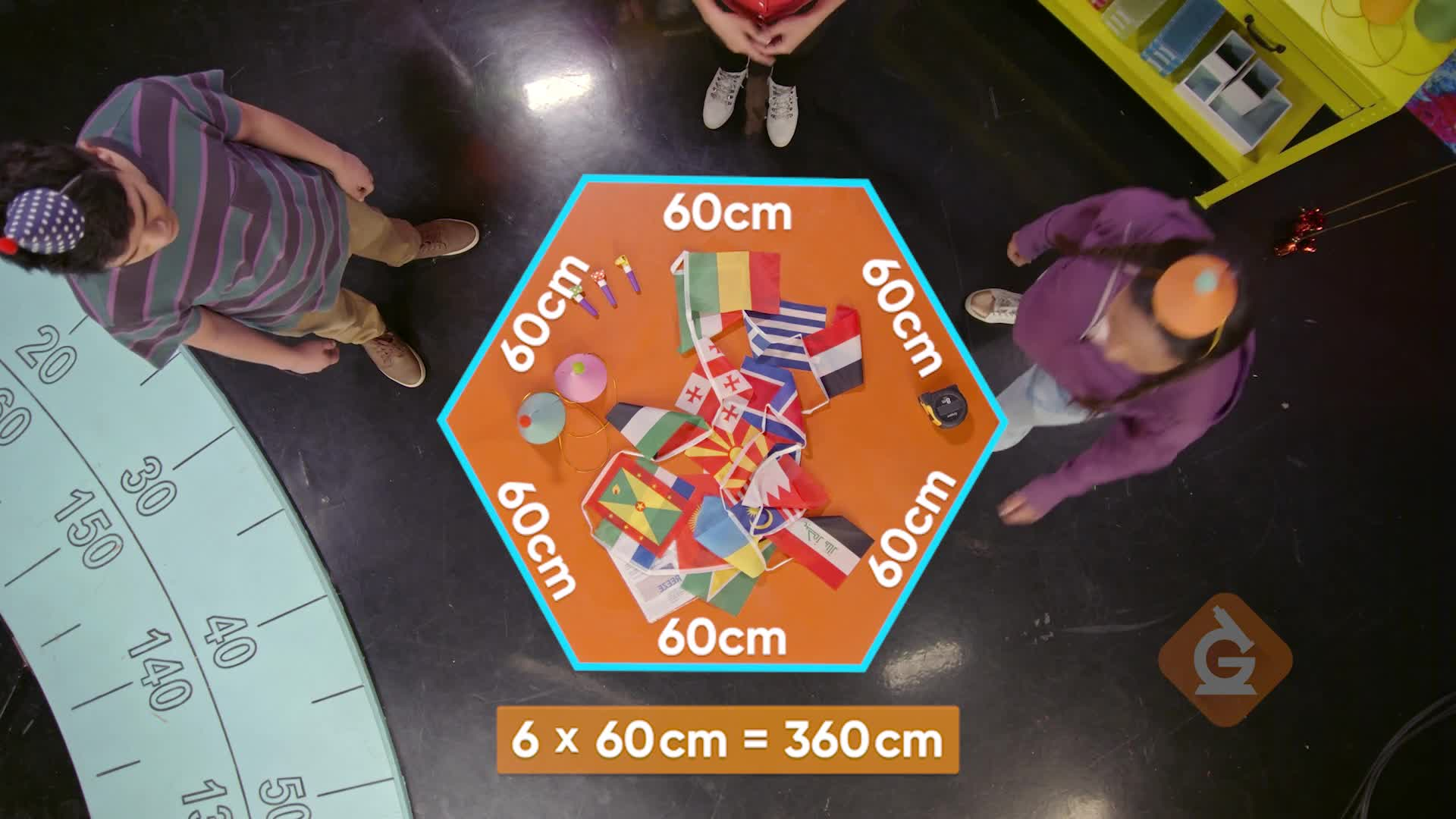WHAT IS PERIMETER?

The distance around a shape, object, or item, is the perimeter. Perimeter is measured in single units, because it is a length.

To better understand perimeter…

WHAT IS PERIMETER?. The distance around a shape, object, or item, is the perimeter. Perimeter is measured in single units, because it is a length. To better understand perimeter…

## LET’S BREAK IT DOWN!

### Around the Puzzles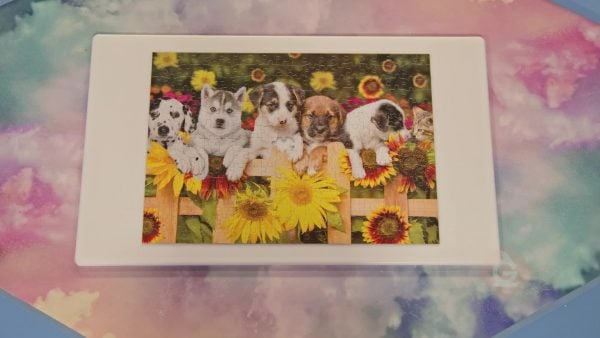Perimeter is the distance around a shape, measuring the “rim” around something. Finding a perimeter can help us when we need to find the length of the outside of something, like a fence, a ribbon, the outside of a puzzle, and many other things! To find perimeter, you measure all of the side lengths and then add the lengths together. Suppose you have a rectangular puzzle that you have completed, and you want to know the total length around the puzzle. This is finding the perimeter. You measure the sides and write them down: 90 cm, 30 cm, 90 cm, 30 cm. Now add to find the total distance around your puzzle. We know that 90 cm + 90 cm = 180 cm, and that 30 cm + 30 cm = 60 cm. Finally, we add 180 cm + 60 cm to get 240 cm. So, the perimeter of your puzzle is 240 cm. Try this one yourself. You want to find the perimeter of your bedroom window. You measure the sides and write them down: 180 cm, 90 cm, 180 cm, 90 cm. What is the perimeter? How did you find it?

Around the Puzzles Perimeter is the distance around a shape, measuring the “rim” around something. Finding a perimeter can help us when we need to find the length of the outside of something, like a fence, a ribbon, the outside of a puzzle, and many other things! To find perimeter, you measure all of the side lengths and then add the lengths together. Suppose you have a rectangular puzzle that you have completed, and you want to know the total length around the puzzle. This is finding the perimeter. You measure the sides and write them down: 90 cm, 30 cm, 90 cm, 30 cm. Now add to find the total distance around your puzzle. We know that 90 cm + 90 cm = 180 cm, and that 30 cm + 30 cm = 60 cm. Finally, we add 180 cm + 60 cm to get 240 cm. So, the perimeter of your puzzle is 240 cm. Try this one yourself. You want to find the perimeter of your bedroom window. You measure the sides and write them down: 180 cm, 90 cm, 180 cm, 90 cm. What is the perimeter? How did you find it?

### Fenced In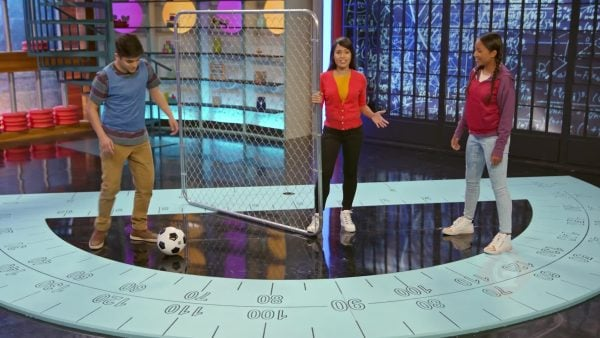Let’s say you want to make a space in your yard for a small L-shaped garden. You plan to build a small fence around the garden. You have 80 meters of fence to use. Your measurements of the sides of the garden are: 9 m, 18 m, 9 m, 9 m, 18 m, and 27 m. You need to add all of the measurements to find the perimeter; so, add 9 m + 18 m + 9 m + 9 m + 18 m + 27 m to get a perimeter of 90 m. You realize that 80 is less than 90, so you do not have enough fencing to go around your garden. Try this one yourself. Suppose you want to fence in a space for a new puppy. You have 100 m of fence to use. The measurements for the space you want to build the fence around are: 10 m, 12 m, 28 m, 11 m, and 30 m. Do you have enough fencing to go around the space where you want to keep your new puppy? Explain how you know.

Fenced In Let’s say you want to make a space in your yard for a small L-shaped garden. You plan to build a small fence around the garden. You have 80 meters of fence to use. Your measurements of the sides of the garden are: 9 m, 18 m, 9 m, 9 m, 18 m, and 27 m. You need to add all of the measurements to find the perimeter; so, add 9 m + 18 m + 9 m + 9 m + 18 m + 27 m to get a perimeter of 90 m. You realize that 80 is less than 90, so you do not have enough fencing to go around your garden. Try this one yourself. Suppose you want to fence in a space for a new puppy. You have 100 m of fence to use. The measurements for the space you want to build the fence around are: 10 m, 12 m, 28 m, 11 m, and 30 m. Do you have enough fencing to go around the space where you want to keep your new puppy? Explain how you know.

### Ruffles and Flags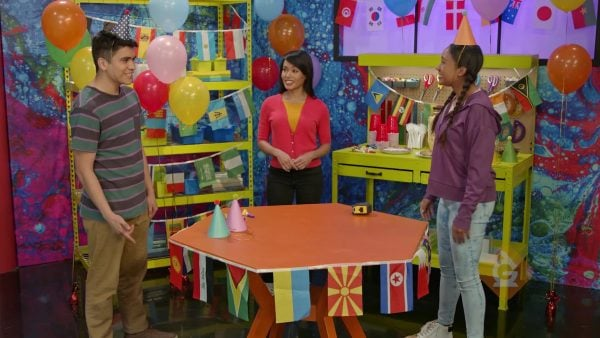Sometimes, when all of the sides are the same length, there could be an easier way to find the perimeter. Let’s say you are helping to decorate for the Flag Day Celebration. You have been asked to find the perimeter of a table that has 5 sides so that you can attach a ruffley flag skirt around the bottom. You know that perimeter is the distance around the table, so you measure the length of each side. The measurements you write down are: 2 m, 2 m, 2 m, 2 m, 2 m. You notice that all 5 sides are the same length! In this case, you can multiply the number of sides by the length of a side, instead of using repeated addition, to find the perimeter. So, you multiply 5 sides by 2 m per side to get 10 m. You need 10 m of the flag skirt to go around the table. Try this one yourself. You have a painting that is in the shape of a hexagon and has 6 sides. You want to put ribbon around the outside of the painting and need to know how much ribbon you will need. You measure and find the lengths to be: 45 cm, 45 cm, 45 cm, 45 cm, 45 cm, 45 cm. After you measure, you realize that all 6 sides are the same. How much ribbon do you need to go around the outside of the painting and how did you find it?

Ruffles and Flags Sometimes, when all of the sides are the same length, there could be an easier way to find the perimeter. Let’s say you are helping to decorate for the Flag Day Celebration. You have been asked to find the perimeter of a table that has 5 sides so that you can attach a ruffley flag skirt around the bottom. You know that perimeter is the distance around the table, so you measure the length of each side. The measurements you write down are: 2 m, 2 m, 2 m, 2 m, 2 m. You notice that all 5 sides are the same length! In this case, you can multiply the number of sides by the length of a side, instead of using repeated addition, to find the perimeter. So, you multiply 5 sides by 2 m per side to get 10 m. You need 10 m of the flag skirt to go around the table. Try this one yourself. You have a painting that is in the shape of a hexagon and has 6 sides. You want to put ribbon around the outside of the painting and need to know how much ribbon you will need. You measure and find the lengths to be: 45 cm, 45 cm, 45 cm, 45 cm, 45 cm, 45 cm. After you measure, you realize that all 6 sides are the same. How much ribbon do you need to go around the outside of the painting and how did you find it?

### Yield to Me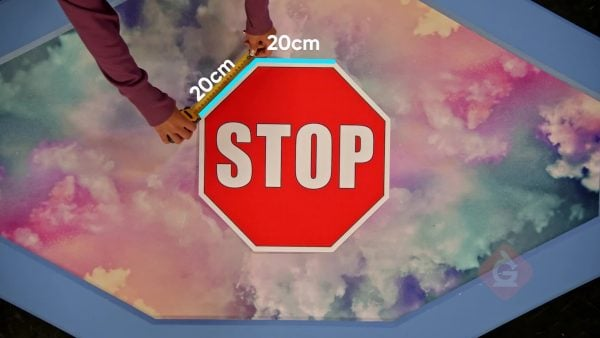The city transportation department wants to put glow-in-the-dark tape around the outside of their traffic signs. You are helping by measuring the perimeters of traffic signs. You start with a Yield Sign, which is a three-sided shape. You measure the sides and write down these measurements: 90 cm, 90 cm, 90 cm. You started to add to find the perimeter, 90 cm + 90 cm = 180 cm; 180 cm + 90 cm = 270 cm. You realize that there was another way you could have found the perimeter because all 3 sides are the same length! You decided to check your addition, and multiplied 3 × 90 cm = 270 cm. You got the same answer both ways! You need 270 cm of tape to go around the outside of the Yield sign. Try this one yourself. Suppose you need to find the perimeter of your bulletin board, so you can put a paper border around it. You measure and write down the following measurements: 60 cm, 60 cm, 60 cm, 60 cm. How would you find the perimeter, and how much border would you need?

Yield to Me The city transportation department wants to put glow-in-the-dark tape around the outside of their traffic signs. You are helping by measuring the perimeters of traffic signs. You start with a Yield Sign, which is a three-sided shape. You measure the sides and write down these measurements: 90 cm, 90 cm, 90 cm. You started to add to find the perimeter, 90 cm + 90 cm = 180 cm; 180 cm + 90 cm = 270 cm. You realize that there was another way you could have found the perimeter because all 3 sides are the same length! You decided to check your addition, and multiplied 3 × 90 cm = 270 cm. You got the same answer both ways! You need 270 cm of tape to go around the outside of the Yield sign. Try this one yourself. Suppose you need to find the perimeter of your bulletin board, so you can put a paper border around it. You measure and write down the following measurements: 60 cm, 60 cm, 60 cm, 60 cm. How would you find the perimeter, and how much border would you need?

## INTRODUCTION TO PERIMETER VOCABULARY

Hexagon
A 6-sided figure.
Pentagon
A 5-sided figure.
Polygon
A plane figure with at least three straight sides and angles.
Perimeter
The distance around a shape.
Regular Polygon
A polygon that has all sides of equal length and all angles equal.
Rectangle
A 4-sided figure with two pairs of equal sides and four right angles.
A 4-sided shape with 4 sides of equal length and four right angles.

## INTRODUCTION TO PERIMETER DISCUSSION QUESTIONS

Perimeter.

### How can you find the perimeter of a square if you only know the length of one side? Why?

You can add the given measurement 4 times because all 4 sides of a square are equal. Or you could multiply the known length by 4, since all 4 sides are the same length.

### A rectangle has one side length of 20 meters and another side length of 10 meters. What are the measurements of the missing sides of the rectangle?

The missing sides are 10 meters and 20 meters.

### An octagon has 8 sides of the same length, 100 cm each. How would you find the perimeter of the octagon?

Add 100 cm eight times; or multiply 8 times 100 cm.

### A pentagon has 4 known side lengths: 5 cm, 15 cm, 20 cm, and 5 cm. It has a perimeter of 58 cm. What is the length of the missing side? How did you find it?

The missing side is 13 cm. "I added the lengths of all sides given, and then subtracted the total from the given perimeter, 58 cm."
X

## Success

We’ve sent you an email with instructions how to reset your password.
Ok

## Choose Your Free Trial Period### 7 DaysContinue to Lessons### 90 DaysGet 90 days free by inviting other teachers to try it too.

Share with Teachers

## Get 90 Days Free

### By inviting 4 other teachers to try it too.

4 required

Skip, I will use a 7 days free trial

## Thank You!

Enjoy your free 90 days trial